# GED Math : Mean

## Example Questions

← Previous 1 3 4

### Example Question #1 : Mean

Find the mean of the following numbers: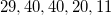Explanation: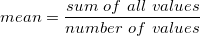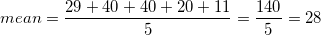### Example Question #2 : Mean

Find the mean of the set of numbers below: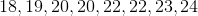Explanation: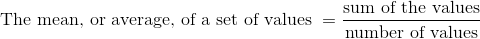In this list,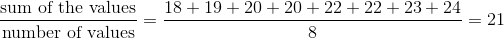### Example Question #1 : Mean

Given the data set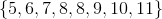, which of the following quantities are equal to each other/one another?

I: The mean

II: The median

III: The mode

II and III only

I and III only

I, II, and III

I and II only

I, II, and III

Explanation:

The mean of a data set is the sum of its elements divided by the number of elements, which here is 8: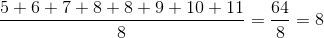The median of a data set with an even number of elements is the mean of the middle two values when the set is arranged in ascending order; both of the middle elements are 8, so the median is 8.

The mode of a data set is the most frequently occurring element. Here, only 8 appears twice, so it is the mode.

All three are equal.

### Example Question #4 : Mean

Veronica scored a 75 and a 71 on her first two exams. To raise her mean score to an 80, what grade does she need to earn on her third test?

94

90

73

85

80

94

Explanation:

The mean score is the sum of all scores divided by the number of scores.

First, find the sum of all three test scores, then divide by the number of tests, 3.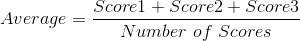If Veronica wants her average to be 80, the equation becomes: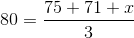We need to solve for.

First, multiply both sides of the equation by 3: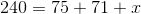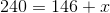Subtract 146 from both sides: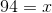Veronica must score a 94 on her third test in order to bring her mean score to 80.

### Example Question #4 : Mean

Veronica went to the mall and bought several small gifts for her sister's birthday. The prices of the items were the following:

$8,$10, $6,$3, $7,$10, $2,$5, $3 What is the mean price? Possible Answers:$10

$3$6

$5$8

$6 Explanation: The mean of a set is the sum of all elements divided by the number of elements in the set: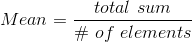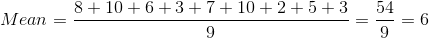The mean is$6.

### Example Question #6 : Mean

On the first two tests of the year, James scored 100 and 70, respectively. He then scored a 96 on his third test. There is just one test remaining, and James wants to finish the semester with a 90 average. What score does he need to receive on the fourth test of the semester to finish with a mean score of 90?

99

90

92

94

100

94

Explanation:

The mean in a data set of scores is the sum of all scores divided by the number of scores: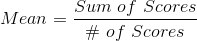Call the fourth test score. Plug in what we know and solve for: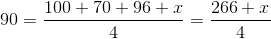Multiply both sides by 4: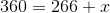Then, subtract 266 from both sides:.To finish with a mean of 90, James must score a 94 on his fourth test.

### Example Question #7 : Mean

Calculate the mean of the following numbers: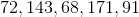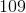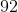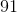Explanation: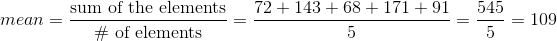### Example Question #8 : Mean

John would like to have a 90 average for math for this semester. John received a 78, 89, 100 on three of his tests. What is the lowest grade he could receive on his last test in order for him to get a 90 average for the semester?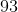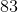Explanation:

In order for John to maintain a 90 average for the marking period, his total for four tests must be 360.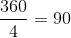or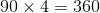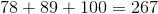If you take the total of 267, which is the total amount of points for the three tests already taken and subtract it from the 360 points which is the total amount of points needed for a 90 average, he would need to get a 93 on his last test.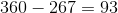### Example Question #3 : Mean

Determine the mean of the numbers: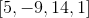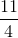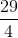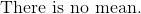Explanation:

The mean is the average of all the numbers in the set of data.

Add the numbers together and divide the sum by 4.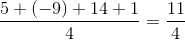The answer is:### Example Question #4 : Mean

Determine the mean of the numbers: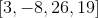Explanation:

The mean is the average of all the number ins the data set.

Add all the numbers and divide the sum by four.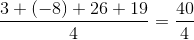The answer is:← Previous 1 3 4